Example Questions

Example Question #4 : How To Find The Equation Of A Perpendicular Line

The endpoints of line segment AB are located at (5, –2) and (–3, 10). What is the equation of the line that is the perpendicular bisector of AB?

3x – 4y = –4

2x – 3y = –10

3x – 4y = –13

2x – 3y = –20

2x + 3y = 14

2x – 3y = –10

Explanation:

We are asked to find the equation of the line that is the perpendicular bisector of AB. If we find a point that the line passes through as well as its slope, we can determine its equation. In order for the line to bisect AB, it must pass through the midpoint of AB. Thus, one point on the line is the midpoint of the AB. We can use the midpoint formula to determine the midpoint of AB with endpoints (5, –2) and (–3, 10).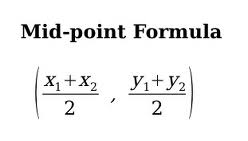The x-coordinate of the midpoint is located at (5 + –3)/2 = 1.

The y-coordinate of the midpoint is located at (–2 + 10)/2 = 4.

Thus, the midpoint of AB is (1, 4).

So, we know that the line passes through (1,4). Now, we can use the fact that the line is perpendicular to AB to find its slope. The product of the slopes of two line segments that are perpendicular is equal to –1. In other words, if we multiply the slope of the line by the slope of AB, we will get –1.

We can use the slope formula to find the slope of AB.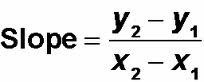slope of AB = (10 – (–2))/(–3 – 5) = 12/–8 = –3/2.

Since the slope of the line multiplied by –3/2 must equal –1, we can write the following:

(slope of the line)(–3/2) = –1

If we multiply both sides by –2/3, we will find the slope of the line.

The slope of the line = (–1)(–2/3) = 2/3.

Thus, the line passes through the ponit (1, 4) and has a slope of 2/3.

We will now use point-slope form to determine the line's equation. Let's let m represent the slope and (x1, y1) represent a ponit on the line.

y – y = m(x – x1)

y – 4 = (2/3)(x – 1)

Multiply both sides by 3 to get rid of the fraction.

3(y – 4) = 2(x – 1)

Distribute both sides.

3y – 12 = 2x – 2

Subtract 3y from both sides.

–12= 2x – 3y – 2

–10 = 2x – 3y.

The equation of the line is 2x – 3y = –10.

The answer is 2x – 3y = –10.

Example Question #5 : How To Find The Equation Of A Perpendicular Line

A line passes through (2, 8) and (4, 15). What is a possible equation for a line perpendicular to this one?

y = (2/7)x + 4

y = (8/3)x + 4

y = (–7/2)x + 4

y = (–2/7)x + 4

y = (–2/7)x + 4

Explanation:

Remember, perpendicular lines have opposite-reciprocal slopes; therefore, let's first find the slope of our line. That is found by the equation: rise/run or y2 – y1/x2 – x1

Substituting in our values: (15 – 8)/(4 – 2) = 7/2

The perpendicular slope is therefore –2/7.

Since ANY perpendicular line will intersect with this line at some point. We merely need to choose the answer that has a line with slope –2/7. Following the slope intercept form (y = mx + b), we know that the coefficient of x will give us this; therefore our answer is: y = (–2/7)x + 4

Example Question #1 : How To Find The Equation Of A Perpendicular Line

Line p is given by the equation y = –x + 4. Which of the following equations describes a line that is perpendicular to p?

x + y = –4

y = –x – 4

x + y = 4

y = –4

y = x + 4

y = x + 4

Explanation:

The equation of line p is given in the form y = mx + b, where m is the slope and b is the y-intercept. Because the equation is y = –x + 4, the slope is m = –1.

If two lines perpendicular, then the product of their slopes is equal to –1. Thus, if we call n the slope of a line perpendicular to line p, then the following equation is true:

m(n) = –1

Because the slope of line p is –1, we can write (–1)n = –1. If we divide both sides by –1, then n = 1. In short, the slope of a line perpendicular to line p must equal 1. We are looking for the equation of a line whose slope equals 1.

Let's examine the answer choices. The equation y = –x – 4 is in the form y = mx + b (which is called point-slope form), so its slope is –1, not 1. Thus, we can eliminate this choice.

Next, let's look at the line x + y = 4. This line is in the form Ax + By = C, where A, B, and C are constants. When a line is in this form, its slope is equal to –A/B. Therefore, the slope of this line is equal to –1/1 = –1, which isn't 1. So we can eliminate x + y = 4. Simiarly, we can eliminate the line x + y = –4.

The line y = –4 is a horizontal line, so its slope is 0, which isn't 1.

The answer is the line y = x + 4, because it is the only line with a slope of 1.

The answer is y = x + 4.

Example Question #7 : How To Find The Equation Of A Perpendicular Line

Give the equation of a line perpendicular to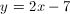.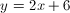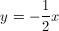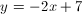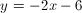Explanation:

The slope of a perpendicular line is the opposite reciprocal, therefore we are looking for a line with a slope of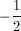.is the only answer choice that satisfies this criteria.

Example Question #8 : How To Find The Equation Of A Perpendicular Line

Find the equation of the line that is perpendicular to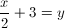and passes through (5, 6).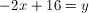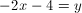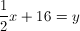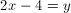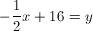Explanation:

We know that the slope of the original line is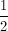Thus the slope of the perpendicular line is the negative reciprocal of, or –2.

Then we plug the slope and point (5, 6) into the form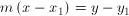, which yields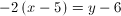When we simplify this, we arrive atExample Question #9 : How To Find The Equation Of A Perpendicular Line

What line is perpendicular to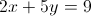through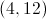?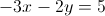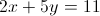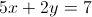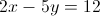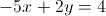Explanation:

We need to find the slope of the given equation by converting it to the slope intercept form: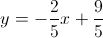The slope is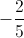and the perpendicular slope would be the opposite reciprocal, or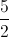The new equation is of the form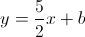and we can use the pointto calculate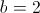. The next step is to convert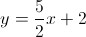into the standard form of.

Example Question #10 : How To Find The Equation Of A Perpendicular Line

What line is perpendicular to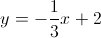and passes through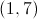?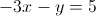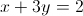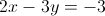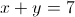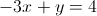Explanation:

Perpendicular slopes are opposite reciprocals.  The original slope is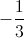so the new perdendicular slope is 3.

We plug the pointand the slope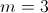into the point-slope form of the equation: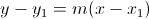to get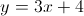or in standard form.

Example Question #11 : How To Find The Equation Of A Perpendicular Line

Solve the system of equations for the point of intersection.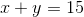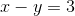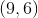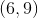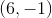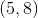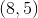Explanation:

First one needs to use one of the two equations to substitute one of the unknowns.

From the second equation we can derive that y = x – 3.

Then we substitute what we got into the first equation which gives us: x + x – 3 = 15.

Next we solve for x, so 2x = 18 and x = 9.

x – y = 3, so = 6.

These two lines will intersect at the point (9,6).

Example Question #12 : How To Find The Equation Of A Perpendicular Line

Line A is perpendicular to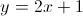and passes the point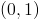. Find the-intercept of line A.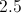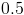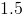Explanation:

We are given an equation of a line and told that line A is perpendicular to it.  The slope of the given line is 2.  Therefore, the slope of line A must be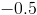, since perpendicular lines have slopes that are negative reciprocals of each other.

The equation for line A will therefore take the form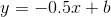, where b is the y-intercept.

Since we are told that it crosses, we can plug in the point and solve for c: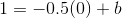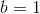Then the equation becomes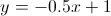.

To find the x-intercept, plug in 0 for y and solve for x: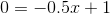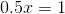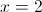Example Question #13 : How To Find The Equation Of A Perpendicular Line

What line is perpendicular to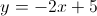through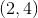?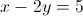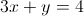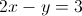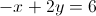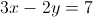Explanation:

The slope of the given line is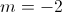, and the slope of the perpendicular line is its negative reciprocal,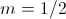.  We take the new slope and the given point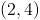and plug them into the slope-intercept form of a line,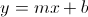.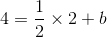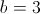Thus, the perpendicular line has the equation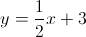, or in standard form,.